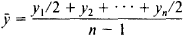# time series

(redirected from Time-series)
Also found in: Dictionary, Thesaurus, Financial.

## time series

[′tīm ‚sir·ēz]
(statistics)
A statistical process analogous to the taking of data at intervals of time.

## time series

ideally, any set of data in which ‘a well-defined quantity is recorded at successive equally-spaced time points over a specific period’ (C. Marsh, 1988), e.g. the RETAIL PRICE INDEX. Where the data fail to fulfil all of these strict criteria, e.g. inadequately standardized variables, or gaps in the series, where the recording interval is not equally spaced, one may still speak of a time series if data over time are involved. However, the problems of interpretation of such a series will be much greater. An important source of time-series data is the CENSUS.

## Time Series

an ordered set of statistical data that characterizes the change or development of a socioeconomic phenomenon over time. An example of a time series is presented in Table 1, which gives data on the production of electric power in the USSR during the period from 1928 to 1973.

The statistical data arranged sequentially in time are called the levels of the time series. The data should be comparable to one another, especially with respect to the territory covered,

Table 1. Production of electric power in the USSR from 1928 to 1973 (billions of kilowatt-hours)
1928 ..............5.0
1932 ..............13.5
1937 ..............36.2
1940 ..............48.3
1950 ..............91.2
1960 ..............292
1970 ..............741
1973 ..............915

range of objects embraced, methods of calculation, critical date, and structure. In an integral time series, the levels characterize the magnitude of the phenomena within certain intervals of time; in a moment time series, the phenomena are characterized at a certain date. The analysis of a time series involves several steps: the determination of the rate and intensity of development of the phenomenon under consideration, the finding of the basic trend of development, the measurement of the variability of the levels, the establishment of relationships with the development of other phenomena, and the performance of a comparative analysis of the development in different countries or regions.

The following statistics are defined for the analysis of dynamic series: absolute increases, rates of growth and increase, average levels of the series, average absolute increases, and average rates of growth and increase. The absolute increase is the difference between one level and the next, and the rate of growth is the ratio of the two levels. When expressed as a coefficient, the rate of increase is the difference between the rate of growth and unity; the rate of increase may also be expressed in percent. The average level of a series for interval series is defined as the arithmetic mean; for moment series it is defined by the formulawhere is the average level, y1 is the initial level, yn is the final level, and η is the number of levels. The average absolute increase is defined as the quotient of the absolute increase for the entire period divided by the number of time units in the period. The average rate of growth can be computed in two ways: either as the geometric average of the rates of growth for the individual intervals of time or as the kth root of the growth rate for the entire period, where k is the number of time units in the period.

The trend is determined by smoothing. The variability of levels of a time series is measured by the mean square of the deviations of the actual levels from the trend. Relationships between the development of the given phonemenon and other phenomena are established by the method of time series correlation, which differs from the conventional correlation method by the possibility of autocorrelation, autoregression, variable correlation, and time lag. Different countries or regions are often analyzed comparatively through the use of a common basis: growth rates are determined for two or more countries for identical time intervals. It is best to calculate the statistics per capita when making a comparative analysis of development. The comprehensive analysis of time series permits identification of the patterns of development of the phenomena reflected in the series.

G. S. KIUDISHEV

References in periodicals archive ?
Air pollution and mortality in elderly people: a time-series study in Sao Paulo, Brazil.
2002)--that using parametric functions in the time-series analyses of air pollution minimizes the bias issue--one possible solution to the bias problem in spatial GAMs is to devise a way to use parametric functions of location.
In time-series studies, a common value of daily exposure is assigned to all subjects residing in a single community, and variations in exposure between subjects are omitted.
Time-series analysis has generally been regarded as suitable for identifying only short-term losses.
In brief, the most suitable model for first difference time-series was determined as ARIMA (0,1,1), also called the first degree integrated moving average model, under the time series investigation.
This paper focusses on the impact of environment mass loading on GPS time-series of position changes and noise characteristic.
This approach allowed the researchers to use previously developed sequence-matching algorithms to search for anomalies and patterns (see Transell, "The Use of Bioinformatics Techniques to Perform Time-Series Trend Matching and Prediction").
Time-series models are models used to study predictions based on the matter of time and to reveal the internal relations of matters.
Auto-Regressive Integrated Moving Average models have been fitted to fish density and biomass time-series of atherinopsids and barred splitfin from Lake Chapala (Becerra-Munoz et al.
today announced that it has developed deep learning technology that can analyze time-series data with a high degree of accuracy.

Site: Follow: Share:
Open / Close